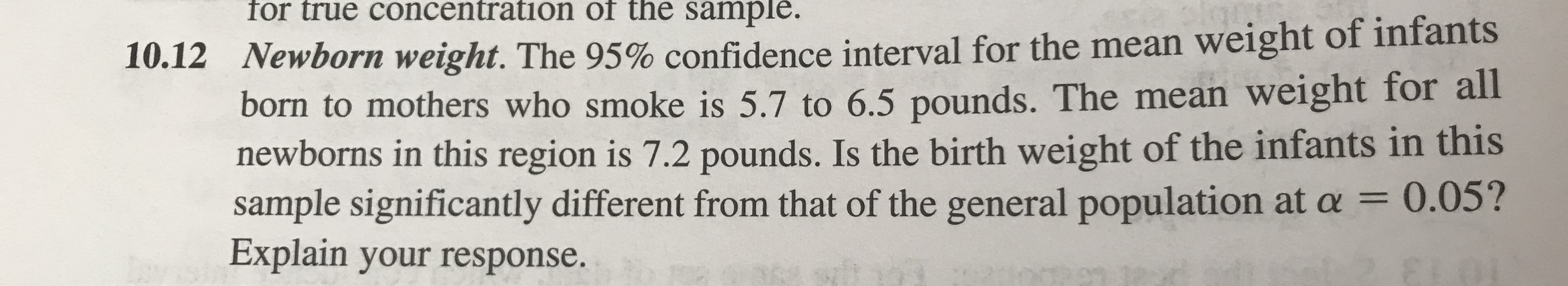# for true concentration of the sample.Newborn weight. The 95% confidence interval for the mean weight of infants1012born to mothers who smoke is 5.7 to 6.5 pounds. The mean weight for allnewborns in this region is 7.2 pounds. Is the birth weight of the infants in thissample significantly different from that of the general population at α = 0.05?Explain your response.

Question
353 views

Is the birth rate of the infants in this sample significantly different from that of the general population at alpha level of 0.05? Explain your response.help_outlineImage Transcriptionclosefor true concentration of the sample. Newborn weight. The 95% confidence interval for the mean weight of infants 1012 born to mothers who smoke is 5.7 to 6.5 pounds. The mean weight for all newborns in this region is 7.2 pounds. Is the birth weight of the infants in this sample significantly different from that of the general population at α = 0.05? Explain your response. fullscreen
check_circle

Step 1

Confidence Interval:

Confidence interval is the interval estimate of the population parameter. It is the range where the population parameter value will lie in between.

The form of the confidence interval is,

CI = Point estimate ± Margin of error

The 100*(1–α)% confidence level means 100*(1–α)% of all possible sample values within the confidence interval will have the population parameter value and 100*α%  of sample values within the confidence interval will not have the population parameter.

The approximate 100*(1–α)% confidence interval for the population mean will be obtained using the formula given below:

Step 2

Check whether the birth rate of infants in the given sample significantly different from that of the general population:

Denote the population mean as μ.

Given that, the population mean weight of infants born to mothers who smoke is μ = 7.2 pounds.

The hypotheses are given below:

Null hypothesis:

H0 : μ = 7.2 pounds.

That is, the population mean weight of infants born to mothers who smoke is equal to 7.2 pounds.

Alternative hypothesis:

H1 : μ ≠ 7.2 pounds (Two tail test).

That is, the population mean weight of infants born to mothers who smoke is not equal to 7.2 pounds.

The 95% confidence interval for the population mean weight of infants ...

### Want to see the full answer?

See Solution

#### Want to see this answer and more?

Solutions are written by subject experts who are available 24/7. Questions are typically answered within 1 hour.*

See Solution
*Response times may vary by subject and question.
Tagged in

### Other